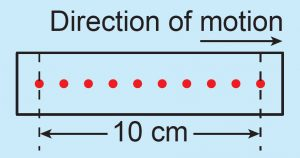# Formative Practice 11.1 – Form 4 Science (KSSM) Chapter 11

Question 1:
State the meaning of the following terms:
(a) Distance
(b) Displacement
(c) Velocity
(d) Acceleration

(a) Distance: Total length of path travelled by an object

(b) Displacement: Distance of the shortest path that connects two locations in a certain direction.

(c) Velocity: Rate of change of displacement

(d) Acceleration: Rate of change of velocity

Question 2:The figure shows a strip of ticker tape that was pulled through a ticker timer vibrating at 50 ticks per second. What is the displacement and velocity shown by the strip of ticker tape?